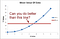# Mean Absolute Error, L1 loss

it is calculated the average of the absolute difference between actual and predicted data point or y or output.

Robust to outliers, handle it pretty well.

computationally expensive because of modulus operator.

there maybe local minima in gradient descent or other optimizer.

# Mean Squared Error, L2 loss, Quadratic loss

it is mostly common used loss function.

Mean Squared Error is the sum of squared distance between actual and predicted output.

MSE is always positive value or 0 as it calculate square.

it is a risk function because it always calculate deviation of actual and predicted value which is going to be squared and average.

it is in the form of quadratic function . so, in the gradient descent, there will be only one global minima, no local minima.

penalizing model by making larger errors by squaring them.

outlier are not handled properly as error become larger.

# Hubar loss

it is combination of both MSE and MAE.

it use MAE, when error is so small it become MSE.

how small that error has to be is dependent on delta(𝛿) parameter which can be tuned.

it is less sensitive to outliers in data than MSE.

outliers are handle properly.

local minima situation is handled here.

might need to train hyperparameter delta(𝛿) which is iterative process.

# R_squared

R-squared (R^2) is a statistical measure that represents the proportion of the variance for a dependent variable that’s explained by an independent variable or variables in a regression model.

if you don’t understand this statement don’t worry, let me tell you in simple words. it basically tells “goodness of best fit line”.

SSres = sum of squares of residuals(error)

SStot = total sum of squaresfor r2<0. red line is average line and blue line is best fit line.

there will be two line. first one is best fit line and second is average line.

R_square became less than 0 when our best line is worse than average line.

R_square is near to 1 means our line is best.

there is one problem here, when number of feature increase, SSres will decrease and it always makes R_squared increase.

predictors means independent features.

r2 = R_square

[(1-r2)(n-1)/ N-p-1] -> (1)

whenever we have so many number of predictors, (N-p-1) will try to decrease and it make (1) to increase and that makes R2_adj to decrease.

if we have low correlated independent features, that time our adj_r2 will decrease.

if our independent features are highly correlated than our r2 will increase that makes (1-r2) decrease and if [(N-1)/(N-p-1)] is higher value it will gonna decrease that makes adj_r2 increase.

That’s all. Thanks for reading this blog :)

smiling...